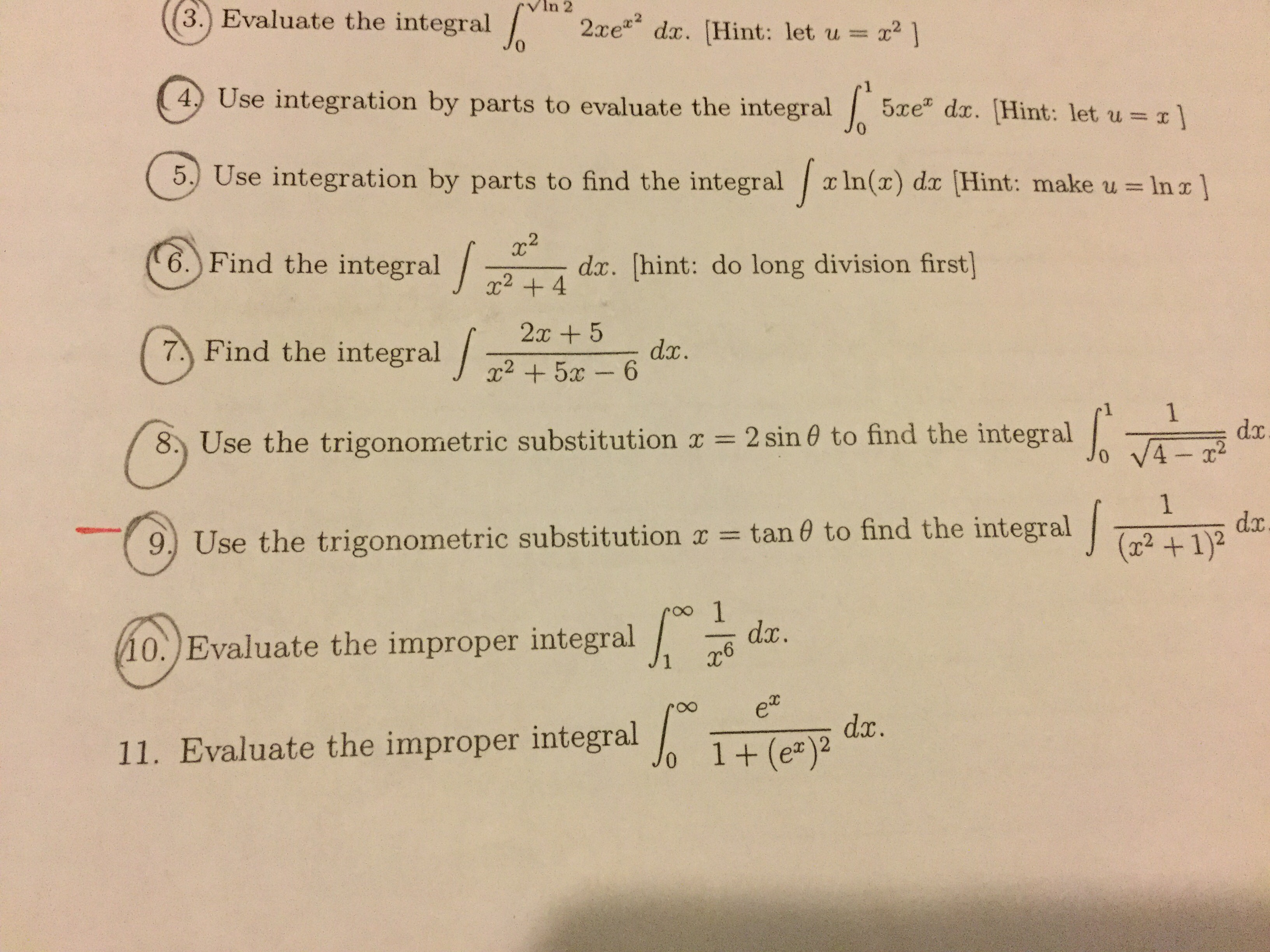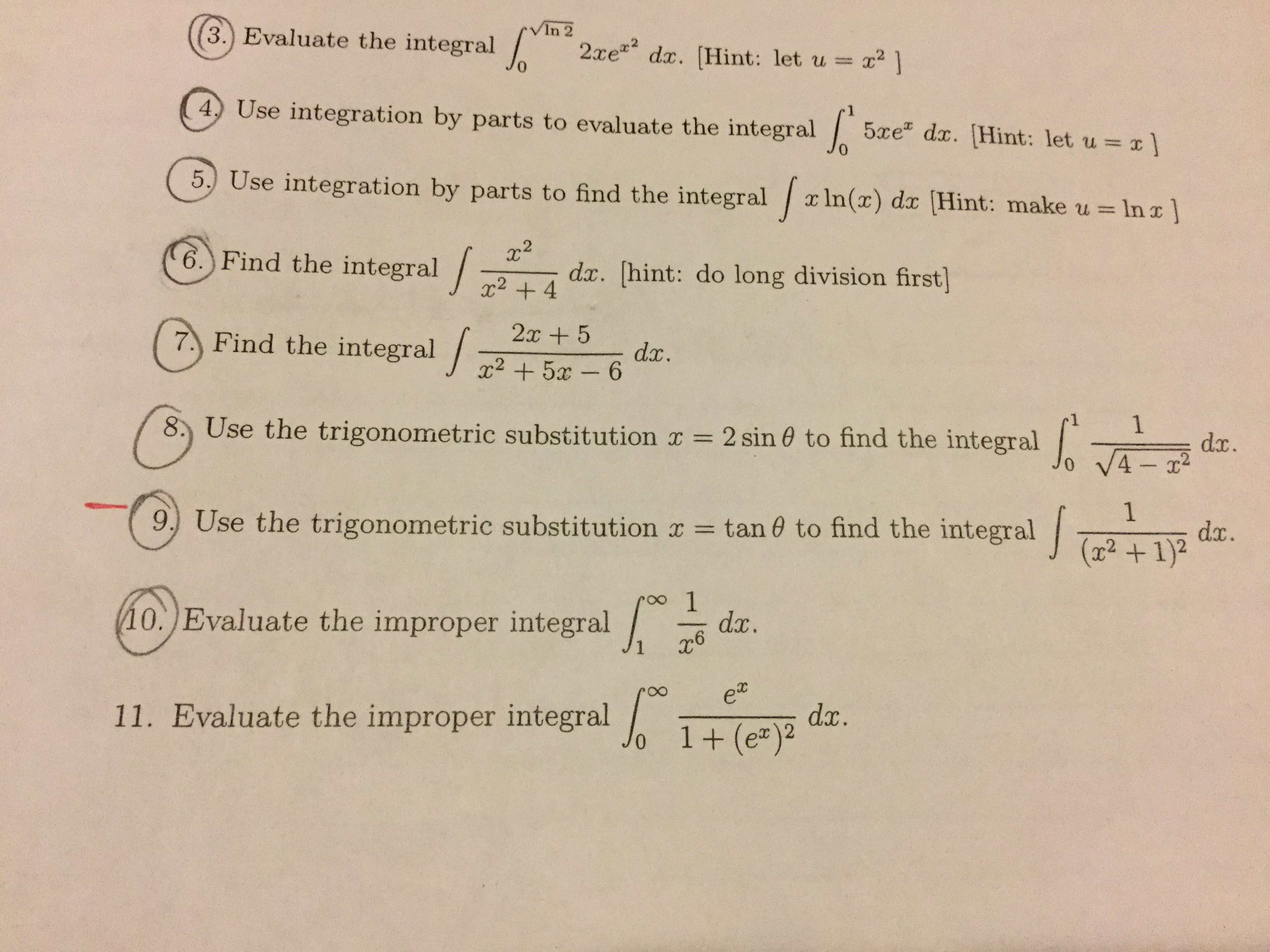# In 2(3.) Evaluate the integral 2xe dx. [Hint: let u x24 Use integration by parts to evaluate the integral5xe dx. Hint: let u ]5 Use integration by parts to find the integral xIn(x) dx [Hint: make u = In r ]22dx. [hint: do long division first]6. Find the integral +42x57 Find the integral 2 + 5x-dx.1dx0 V4-r28. Use the trigonometric substitution x= 2 sin 0 to find the integralamd1dx(x21)29) Use the trigonometric substitution x = tan 0 to find the integral10.)Evaluate the improper integral dx.dx.1+(e)211. Evaluate the improper integral (3.) Evaluate the integral 2xe dx. [Hint: let u x2 ]VIn 24 Use integration by parts to evaluate the integral5xe dx. [Hint: let u x]5. Use integration by parts to find the integral ln (x) dx [Hint: make u-= In x ]6. Find the integral + 4dx. [hint: do long division first2x57 Find the integraldx.x2 5x-68. Use the trigonometric substitution x = 2 sin 0 to find the integral1dx.0 V4-219 Use the trigonometric substitution x tane to find the integraldx.(xr21)210. )Evaluate the improper integraldx.alen111. Evaluate the improper integral Jo 1+ (e)2e0dx.-19

Question

Can you help me on #9?help_outlineImage TranscriptioncloseIn 2 (3.) Evaluate the integral 2xe dx. [Hint: let u x2 4 Use integration by parts to evaluate the integral 5xe dx. Hint: let u ] 5 Use integration by parts to find the integral xIn(x) dx [Hint: make u = In r ] 22 dx. [hint: do long division first] 6. Find the integral +4 2x5 7 Find the integral 2 + 5x- dx. 1 dx 0 V4-r2 8. Use the trigonometric substitution x= 2 sin 0 to find the integral amd 1 dx (x21)2 9) Use the trigonometric substitution x = tan 0 to find the integral 10.)Evaluate the improper integral dx. dx. 1+(e)2 11. Evaluate the improper integral fullscreenhelp_outlineImage Transcriptionclose(3.) Evaluate the integral 2xe dx. [Hint: let u x2 ] VIn 2 4 Use integration by parts to evaluate the integral 5xe dx. [Hint: let u x] 5. Use integration by parts to find the integral ln (x) dx [Hint: make u- = In x ] 6. Find the integral + 4 dx. [hint: do long division first 2x5 7 Find the integral dx. x2 5x-6 8. Use the trigonometric substitution x = 2 sin 0 to find the integral 1 dx. 0 V4-2 1 9 Use the trigonometric substitution x tane to find the integral dx. (xr21)2 10. )Evaluate the improper integral dx. alen 1 11. Evaluate the improper integral Jo 1+ (e)2 e0 dx. -19 fullscreen
check_circle

Step 1

Find integ...

### Want to see the full answer?

See Solution

#### Want to see this answer and more?

Solutions are written by subject experts who are available 24/7. Questions are typically answered within 1 hour.*

See Solution
*Response times may vary by subject and question.
Tagged in

### Integration